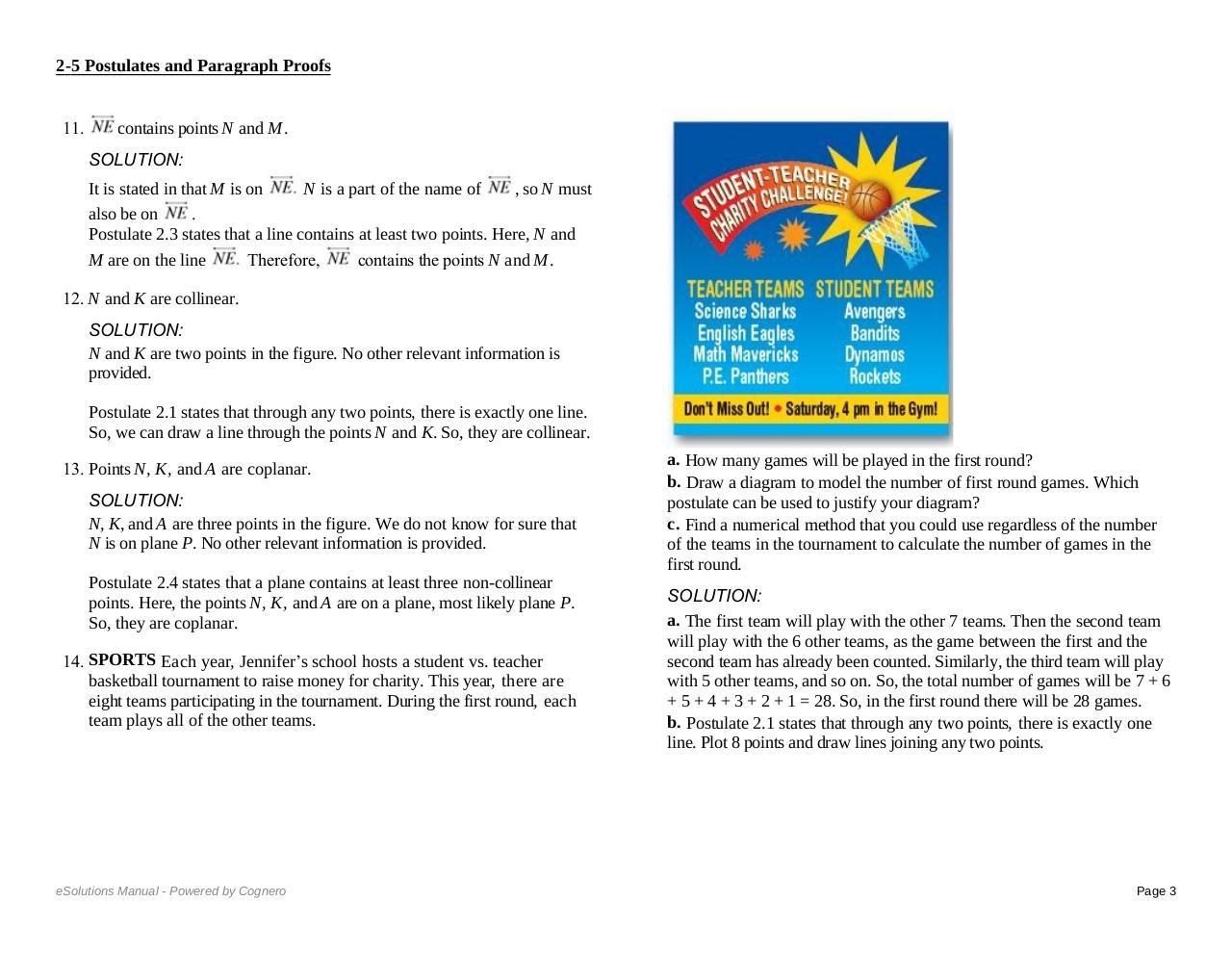# 2 5ALL.pdfPage 1 2 34518

#### Text preview

M, K, and N are all points and they are not collinear.
Postulate 2.2 states that through any three noncollinear points, there is
exactly one plane. So, there exist a plane through the points M, K, and N.
2-5 Postulates
andNParagraph
Proofs
So, M, K, and
are coplanar.
11.

So, they are coplanar.
14. SPORTS Each year, Jennifer’s school hosts a student vs. teacher
basketball tournament to raise money for charity. This year, there are
eight teams participating in the tournament. During the first round, each
team plays all of the other teams.

contains points N and M .
SOLUTION:
It is stated in that M is on
N is a part of the name of
, so N must
also be on

Postulate 2.3 states that a line contains at least two points. Here, N and
M are on the line
Therefore,
contains the points N and M .

12. N and K are collinear.
SOLUTION:
N and K are two points in the figure. No other relevant information is
provided.

Postulate 2.1 states that through any two points, there is exactly one line.
So, we can draw a line through the points N and K. So, they are collinear.
13. Points N, K, and A are coplanar.
SOLUTION:
N, K, and A are three points in the figure. We do not know for sure that
N is on plane P. No other relevant information is provided.

Postulate 2.4 states that a plane contains at least three non-collinear
points. Here, the points N, K, and A are on a plane, most likely plane P.
So, they are coplanar.
14. SPORTS Each year, Jennifer’s school hosts a student vs. teacher
basketball tournament to raise money for charity. This year, there are
eight teams participating in the tournament. During the first round, each
team plays all of the other teams.

a. How many games will be played in the first round?
b. Draw a diagram to model the number of first round games. Which
postulate can be used to justify your diagram?
c. Find a numerical method that you could use regardless of the number
of the teams in the tournament to calculate the number of games in the
first round.
SOLUTION:
a. The first team will play with the other 7 teams. Then the second team
will play with the 6 other teams, as the game between the first and the
second team has already been counted. Similarly, the third team will play
with 5 other teams, and so on. So, the total number of games will be 7 + 6
+ 5 + 4 + 3 + 2 + 1 = 28. So, in the first round there will be 28 games.
b. Postulate 2.1 states that through any two points, there is exactly one
line. Plot 8 points and draw lines joining any two points.

Page 3

c.  If there are 8 teams in the tournament, the number of games in the
first round is (8 − 1) + (8 − 2) +…+ 1. Therefore, if there are n teams in